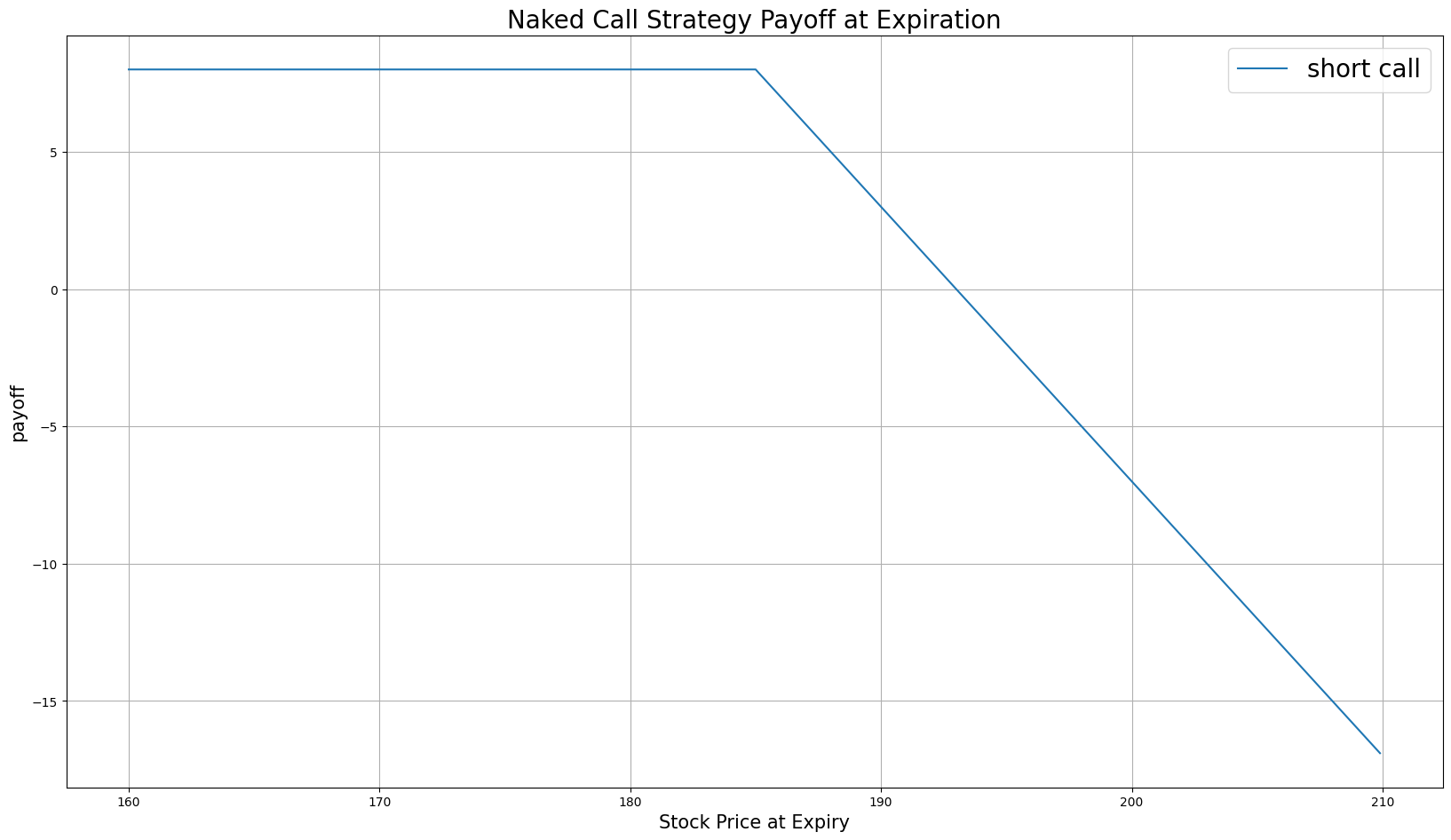# Option Strategies

## Naked Call

### Introduction

A Naked Call, also known as an uncovered call, consists of selling call Options without owning the underlying asset. It's called "naked" because you don't have any cover or protection in the form of owning the underlying asset, which exposes you to potentially unlimited risk. Naked calls aim to profit from the Option premium by selling calls. At any time for American Options or at expiration for European Options, if the stock moves below the strike price, you keep the premium. If the underlying price moves above the strike, the Option buyer can exercise the Options contract, which means you need buy the underlying at market price to fulfill your obligation to sell it at the strike price and keep the premium.

### Implementation

Follow these steps to implement the naked call strategy:

1. In the Initialize method, set the start date, end date, starting cash, and Options universe.
2. private Symbol _call, _symbol;

public override void Initialize()
{
SetStartDate(2014, 1, 1);
SetEndDate(2014, 3, 1);
SetCash(100000);

_symbol = option.Symbol;
option.SetFilter(-3, 3, 0, 31);
}
def Initialize(self) -> None:
self.SetStartDate(2014, 1, 1)
self.SetEndDate(2014, 3, 1)
self.SetCash(100000)

self.symbol = option.Symbol
self.call = None

option.SetFilter(-3, 3, 0, 31)
3. In the OnData method, select the Option contract.
4. public override void OnData(Slice slice)
{
if (_call != null && Portfolio[_call].Invested) return;

if (!slice.OptionChains.TryGetValue(_symbol, out var chain)) return;

// Find ATM call with the farthest expiry
var expiry = chain.Max(x => x.Expiry);
var atmCall = chain
.Where(x => x.Right == OptionRight.Call && x.Expiry == expiry)
.OrderBy(x => Math.Abs(x.Strike - chain.Underlying.Price))
.FirstOrDefault();
def OnData(self, slice: Slice) -> None:
if self.call and self.Portfolio[self.call].Invested:
return

chain = slice.OptionChains.get(self.symbol)
if not chain:
return

# Find ATM call with the farthest expiry
expiry = max([x.Expiry for x in chain])
call_contracts = sorted([x for x in chain
if x.Right == OptionRight.Call and x.Expiry == expiry],
key=lambda x: abs(chain.Underlying.Price - x.Strike))

if not call_contracts:
return

atm_call = call_contracts
5. In the OnData method, call the OptionStrategies.NakedCall method and then submit the order.
6. var nakedCall = OptionStrategies.NakedCall(_symbol, atmCall.Strike, expiry);

_call = atmCall.Symbol;
naked_call = OptionStrategies.NakedCall(self.symbol, atm_call.Strike, expiry)

self.call = atm_call.Symbol

Option strategies synchronously execute by default. To asynchronously execute Option strategies, set the asynchronous argument to Falsefalse. You can also provide a tag and order properties to the Buy method.

Buy(optionStrategy, quantity, asynchronous, tag, orderProperties);

self.Buy(option_strategy, quantity, asynchronous, tag, order_properties)


### Strategy Payoff

The payoff of the strategy is

$$\begin{array}{rcll} C^{K}_T & = & (S_T - K)^{+}\\ P_T & = & (C^{K}_0 - C^{K}_T)\times m - fee \end{array}$$ $$\begin{array}{rcll} \textrm{where} & C^{K}_T & = & \textrm{Call value at time T}\\ & S_T & = & \textrm{Underlying asset price at time T}\\ & K & = & \textrm{Call strike price}\\ & P_T & = & \textrm{Payout total at time T}\\ & C^{K}_0 & = & \textrm{Call price when the trade opened (credit received)}\\ & m & = & \textrm{Contract multiplier}\\ & T & = & \textrm{Time of expiration} \end{array}$$

The following chart shows the payoff at expiration:The maximum profit is $C^{K}_0$, which occurs when the underlying price is at or below the strike price of the call at expiration.

The maximum loss is unlimited because there is no limit to how high the underlying asset's price can rise.

If the Option is American Option, there is risk of early assignment on the sold contract.

### Example

The following table shows the price details of the assets in the algorithm:

AssetPrice ($)Strike ($)
Call3.35185.00
Underlying Equity at expiration190.01-

Therefore, the payoff is

$$\begin{array}{rcll} C^{K}_T & = & (S_T - K)^{+}\\ & = & (190.01 - 185)^{+}\\ & = & 5.01\\ P_T & = & (C^{K}_0 - C^{K}_T)\times m - fee\\ & = & (3.35 - 5.01)\times m - fee\\ & = & -1.66 \times 100 - 2\\ & = & -167 \end{array}$$

So, the strategy loses \$167.

The following algorithm implements a naked call strategy:

You can also see our Videos. You can also get in touch with us via Discord.